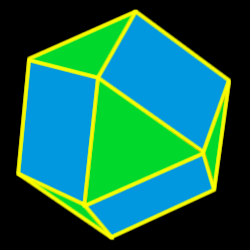# Cuboctahedron

Properties of the cuboctahedron: Number of faces, edges and dihedral angle measure

The cuboctahedron is created by either truncating (cutting off) the
cube one half of the way into each edge or
by truncating the octahedron one half of the way into each side.14 total faces:
8 equilateral triangles and 6 squares

12 vertices:
2 triangles and 2 squares

24 edges

Dihedral angles: 125 degrees, 16 minutes